International
Tables for
Crystallography
Volume B
Reciprocal space
Edited by U. Shmueli

International Tables for Crystallography (2006). Vol. B. ch. 2.4, p. 265   | 1 | 2 |

## Section 2.4.2.2. Single isomorphous replacement method

M. Vijayana* and S. Ramaseshanb

aMolecular Biophysics Unit, Indian Institute of Science, Bangalore 560 012, India, and bRaman Research Institute, Bangalore 560 080, India
Correspondence e-mail:  mv@mbu.iisc.ernet.in

#### 2.4.2.2. Single isomorphous replacement method

| top | pdf |

The number of replaceable (or `added') atoms is usually small and they generally have high atomic numbers. Their positions are often determined by a Patterson synthesis of one type or another (see Chapter 2.3). It will therefore be assumed in the following discussion thatis known. Then it can be readily seen by referring to Fig. 2.4.2.2that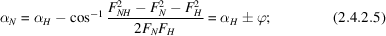whenis derived from its cosine function, it could obviously be positive or negative. Hence, there are two possible solutions for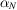. These two solutions are distributed symmetrically about. One of these would correspond to the correct value of. Therefore, in general, the phase angle cannot be unambiguously determined using a pair of isomorphous crystals.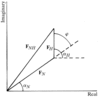Figure 2.4.2.2 | top | pdf |Relationship between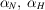and.

The twofold ambiguity in phase angle vanishes when the structures are centrosymmetric.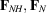andare all real in centric data and the corresponding phase angles are 0 or. From (2.4.2.4)The sign ofis already known and the signs of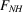andcan be readily determined from (2.4.2.6)(Robertson & Woodward, 1937).

When the data are acentric, the best one can do is to use both the possible phase angles simultaneously in a Fourier synthesis (Bokhoven et al., 1951). This double-phased synthesis, which is equivalent to the isomorphous synthesis of Ramachandran & Raman (1959), contains the structure and its inverse when the replaceable atoms have a centrosymmetric distribution (Ramachandran & Srinivasan, 1970). When the distribution is noncentrosymmetric, however, the synthesis contains peaks corresponding to the structure and general background. Fourier syntheses computed using the single isomorphous replacement method of Blow & Rossmann (1961) and Kartha (1961) have the same properties. In this method, the phase angle is taken to be the average of the two possible solutions of, which is always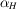or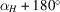. Also, the Fourier coefficients are multiplied by cos, following arguments based on the Blow & Crick (1959) formulation of phase evaluation (see Section 2.4.4.4). Although Blow & Rossmann (1961) have shown that this method could yield interpretable protein Fourier maps, it is rarely used as such in protein crystallography as the Fourier maps computed using it usually have unacceptable background levels (Blundell & Johnson, 1976).

### ReferencesBlow, D. M. & Crick, F. H. C. (1959). The treatment of errors in the isomorphous replacement method. Acta Cryst. 12, 794–802.Google ScholarBlow, D. M. & Rossmann, M. G. (1961). The single isomorphous replacement method. Acta Cryst. 14, 1195–1202.Google ScholarBlundell, T. L. & Johnson, L. N. (1976). Protein crystallography. London: Academic Press.Google ScholarBokhoven, C., Schoone, J. C. & Bijvoet, J. M. (1951). The Fourier synthesis of the crystal structure of strychnine sulphate pentahydrate. Acta Cryst. 4, 275–280.Google ScholarKartha, G. (1961). Isomorphous replacement in non-centrosymmetric structures. Acta Cryst. 14, 680–686.Google ScholarRamachandran, G. N. & Raman, S. (1959). Syntheses for the deconvolution of the Patterson function. Part I. General principles. Acta Cryst. 12, 957–964.Google ScholarRamachandran, G. N. & Srinivasan, R. (1970). Fourier methods in crystallography. New York: Wiley–Interscience.Google ScholarRobertson, J. M. & Woodward, I. (1937). An X-ray study of the phthalocyanines. Part III. Quantitative structure determination of nickel phthalocyanine. J. Chem. Soc. pp. 219–230.Google Scholar